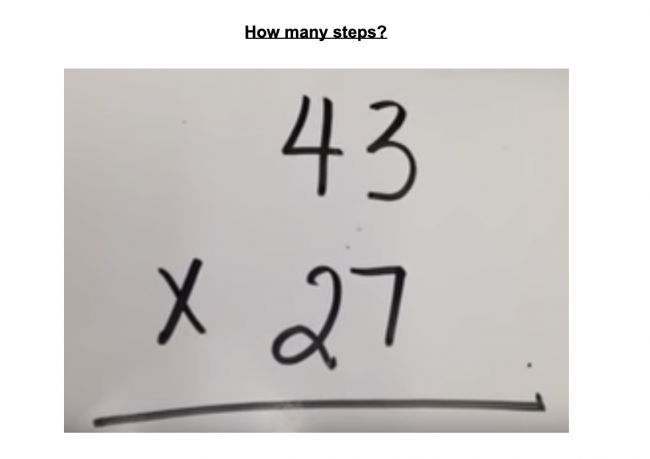# How many steps?

Preview:Students attempt to determine the relationship between how many steps it takes to multiply a pair of numbers and the size of those numbers. This project can either be done as practice for a particular multiplication strategy, to compare different multiplication strategies, or to come up with an algebraic rule for the number of steps based on the size of the two numbers being multiplied.

Project
Big Idea:
Grade 4: Development of computational fluency and multiplicative thinking requires analysis of patterns and relations in multiplication and division.
Grade 9: The principles and processes underlying operations with numbers apply equally to algebraic situations and can be described and analyzed.
Curricular Competency:
Reasoning and analyzing: Use reasoning to explore and make connections
Understanding and solving: Develop, demonstrate, and apply mathematical understanding through play, inquiry, and problem solving
Communicating and representing: Communicate mathematical thinking in many ways
Communicating and representing: Use mathematical vocabulary and language to contribute to mathematical discussions
Communicating and representing: Explain and justify mathematical ideas and decisions